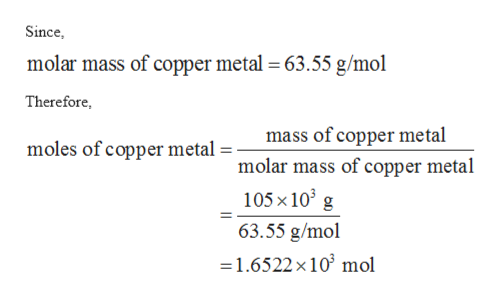# How many copper atoms are in a pure copper statue with a mass of 105kg?

Question
305 views

How many copper atoms are in a pure copper statue with a mass of 105kg?

check_circle

Step 1

The value is given as follows:

Step 2

The number of moles of copper metal can be calculated as follows:help_outlineImage TranscriptioncloseSince molar mass of copper metal = 63.55 g/mol Therefore mass of copper metal molar mass of copper metal moles of copper metal 105 x 10 g 63.55 g/mol 1.6522 x 10 mol fullscreen
Step 3

Since, 1 mol of copper metal contains 6.022 x 1023 atoms. Therefore, total number of copper...

### Want to see the full answer?

See Solution

#### Want to see this answer and more?

Solutions are written by subject experts who are available 24/7. Questions are typically answered within 1 hour.*

See Solution
*Response times may vary by subject and question.
Tagged in

### Chemistry